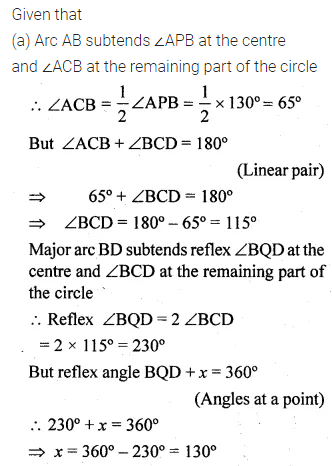Guru

# Question 8. (a) In the figure given below, P and Q are centres of two circles intersecting at B and C. ACD is a st. line. Calculate the numerical value of x.

• 0

This is the basic and conceptual question from Chapter name- circles
Topic – Angle properties of circles
Chapter number- 15

We have been given the figure of circle with certain information about the figure and we have to find the value of x.

ICSE Avichal publication
Understanding ICSE Mathematics
Question 8 (a)

Share